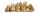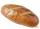# Number with ones

The first digit of the number is 1, if we move this digit to the end we get a 3 times higher number, which is the number?

Result

n =  142857

#### Solution:Leave us a comment of example and its solution (i.e. if it is still somewhat unclear...):

Showing 1 comment:Dr Math
prima cifra a unui numar este 1 daca mutam aceasta cifra la sfarsit obtinem un numar de 3 ori mai mare care este numarul?#### To solve this example are needed these knowledge from mathematics:

Do you have a linear equation or system of equations and looking for its solution? Or do you have quadratic equation?

## Next similar examples:

1. Seven timesWhich number seven times is just as higher as 27, how much is smaller than 29?
2. Unknown number 11That number increased by three equals three times itself?
3. One-thirdA one-third of unknown number is equal to five times as great as the difference of the same unknown number and number 28. Determine the unknown number.
4. Unknown number 6Determine the unknown number, which is by 1.5 greater than its fourth.
5. Dropped sheetsThree consecutive sheets dropped from the book. The sum of the numbers on the pages of the dropped sheets is 273. What number has the last page of the dropped sheets?
6. Last pageTwo consecutive sheets dropped out of the book. The sum of the numbers on the sides of the dropped sheets is 154. What is the number of the last page of the dropped sheets?
7. TwentyTwenty rabbits are put in 4 cells so that there are different number of rabbits in each cell contains at least 3 rabbits. What is the largest possible number of rabbits in one cell
8. Write decimalsWrite in the decimal system the short and advanced form of these numbers: a) four thousand seventy-nine b) five hundred and one thousand six hundred and ten c) nine million twenty-six
9. Find xSolve: if 2(x-1)=14, then x= (solve an equation with one unknown)
10. Find unknownFind unknown numerator: 4/8 + _/8 = 1
11. NormThree workers planted 3555 seedlings of tomatoes in one dey. First worked at the standard norm, the second planted 120 seedlings more and the third 135 seedlings more than the first worker. How many seedlings were standard norm?
12. Simple equationSolve the following simple equation: 2. (4x + 3) = 2-5. (1-x)
13. Equation 29Solve next equation: 2 ( 2x + 3 ) = 8 ( 1 - x) -5 ( x -2 )
14. Simple equationSolve for x: 3(x + 2) = x - 18
15. Negative in equation2x + 3 + 7x = – 24, what is the value of x?
16. Forest nurseryIn the forest nursery after winter, they found that 1/10 stems died out of them. For them, they land 193 new spruces. How many spruces are in the forest nursery?
17. Bread5 of the same bread has the same weight as three bread and 4 kg of fruit. What weight has one bread?